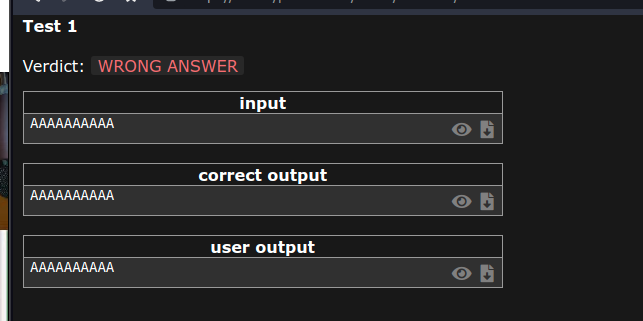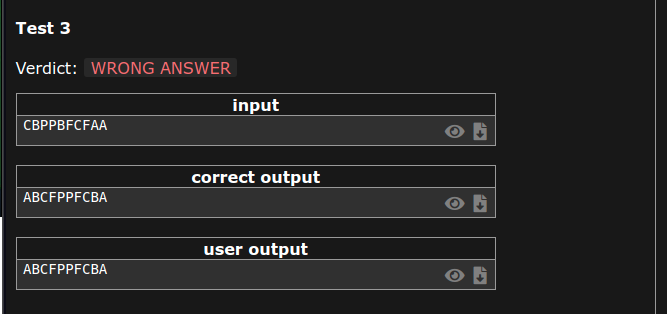Can any help me with the palindrome reorder problem on CSES

Hello all,
I am trying to solve the palindrome partitioning problem in CSES and have written the below code

#include<bits/stdc++.h>
#define endl "\n"
#define ll long long
using namespace std;

int main() {

string str;
cin >> str;

map<char,int> hashmap;

for(char ch: str) {
hashmap[ch]++;
}

int oddCount = 0;

map<char,int>::iterator itr;
for(itr = hashmap.begin(); itr != hashmap.end(); itr++) {
int count = itr->second;

if (count%2 != 0) {
oddCount++;

if (oddCount > 1) {
cout << "NO SOLUTION" ;
return 0;
}
}
}

char oddChar ;

for(itr = hashmap.begin(); itr != hashmap.end(); itr++) {
int currentCount = itr->second;

// if odd count
if (currentCount % 2 != 0) {
oddChar = itr->first;
}

currentCount = currentCount/2;

char currentChar = itr->first;

while(currentCount--) {
}
}Thank you in advance